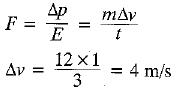# MCQ : Force And Laws Of Motion - 2

## 20 Questions MCQ Test Science Class 9 | MCQ : Force And Laws Of Motion - 2

Description
This mock test of MCQ : Force And Laws Of Motion - 2 for Class 9 helps you for every Class 9 entrance exam. This contains 20 Multiple Choice Questions for Class 9 MCQ : Force And Laws Of Motion - 2 (mcq) to study with solutions a complete question bank. The solved questions answers in this MCQ : Force And Laws Of Motion - 2 quiz give you a good mix of easy questions and tough questions. Class 9 students definitely take this MCQ : Force And Laws Of Motion - 2 exercise for a better result in the exam. You can find other MCQ : Force And Laws Of Motion - 2 extra questions, long questions & short questions for Class 9 on EduRev as well by searching above.
QUESTION: 1

Solution:
QUESTION: 2

Solution:
QUESTION: 3

### Inertia

Solution:
QUESTION: 4

A plate, a ball and a child all have the same mass. The one having more inertia is the

Solution:
QUESTION: 5

A passenger in a moving train tosses a coin which falls behind him. It means that the motion of the train is

Solution:
QUESTION: 6

Two equal masses m each moving in the opposite direction with the same speed v collide and stick to each other. The velocity of the combined mass is

Solution:

Total initial momentum = Total final momentum = zero

QUESTION: 7

The rate of change of momentum with respect to time is measured in

Solution:

We know,  p = mv
Units of p, ⇒ kg ms-1
We have to measure, rate of change of momentum with respect to time ;
⇒  kg m s-2

QUESTION: 8

The incorrect option regarding action-reaction pair is

Solution:

Since, action and reaction forces are equal in magnitude and opposite in directions. Therefore, there resultant is zero.

QUESTION: 9

A goalkeeper in a game of football pulls his hands backwards after holding the ball shot at the goal. This enables the goalkeeper to

Solution:

A large force that acts in a very short duration of time is reffered to as impulsive force. This force which is large due to a high speed such as kick of a football may be disastrous as the momentum change is large and hence a goalkeeper holds his hand backwards in order to reduce the force exerted on his hands.

QUESTION: 10

If force, change in momentum and time are given by F, p and t respectively, then they are related b

Solution:

The rate at which an object’s momentum changes is equal to the force acting on the object. If a force F acts on an object for a time Δt, then the change in the object's momentum is Δp = FΔt.

QUESTION: 11

According to Newton’s third law of motion, action and reaction

Solution:
QUESTION: 12

The minimum number of unequal forces that can make zero resultant is

Solution:

Three vectors of unequal magnitude which can be represented by the three sides of a triangle taken in order, produce zero resultant.

QUESTION: 13

An object of mass 2 kg is sliding with a constant velocity of 4 ms-1 on a frictional horizontal table. The force required to keep the object moving with same velocity is

Solution:

From newton's Ist law of motion, since there is no external force acting on the object it will remain in its original state of motion. Hence, zero force is required to keep the object moving with constant velocity.

QUESTION: 14

A mass M breaks into two pieces in the ratio 1 : 3 while at rest. If the heavier has a speed of v, the speed of the lighter is

Solution:
QUESTION: 15

An object of mass of 2 kg is sliding with a velocity of 4 ms-1 on a frictional horizontal surface. The retarding force necessary to stop the object in 1 second is

Solution:

Here, u = 0 m /s
a  = v- u / t
=>  0-4/1 = > -4.
Formula of regarding force remains same.
F = - ma. ( negative sign comes due to  restarting  acceleration.)
Therefore,  F = - 2* (-4)
F  =  8 N

QUESTION: 16

The two states of motion treated alike by the Newton’s first law, among A, B, C and D are
A : Rest
B : Uniform motion
C : Uniformly accelerated
D : Non-uniformly accelerated

Solution:
QUESTION: 17

Passengers standing in a bus are thrown outwards when the bus takes a turn suddenly. This happens because of

Solution:
QUESTION: 18

When a 12 N force acts on 3 kg mass for a second, the change in velocity is (in m/s)

Solution:QUESTION: 19

A force of ‘P* N acts on a particle so as to accelerate it from rest to a velocity 'v' m/s. The force 'P’ is then replaced by ‘Q’ N which decelerates it to rest.

Solution:

To decelerate a body, a force must be applied opposite to the direction of velocity, irrespective of its magnitude.
According to the condition of motion under two forces P and Q must be acting in opposite directions and may be equal, not necessarily must be equal.

QUESTION: 20

Which of the following does not happen? As an automobile accelerates suddenly,

Solution:

When a vehicle accelerates and suddenly applies a break we possess what we call inertia. And when it suddenly applies a break we tend to retain that Velocity so we fall backwards thus satisfying the law of inertia or what is also called Newton's first law of motion.